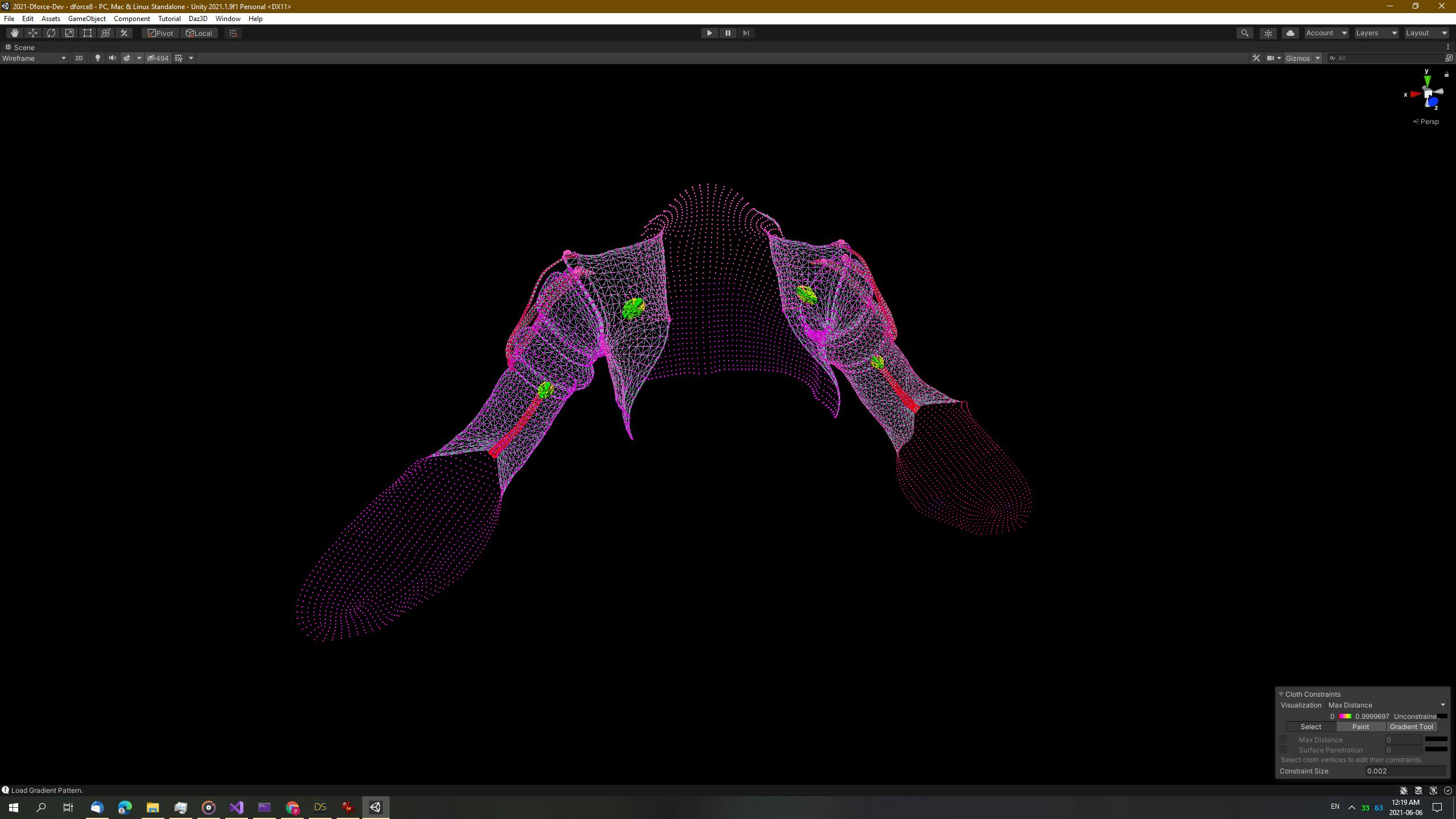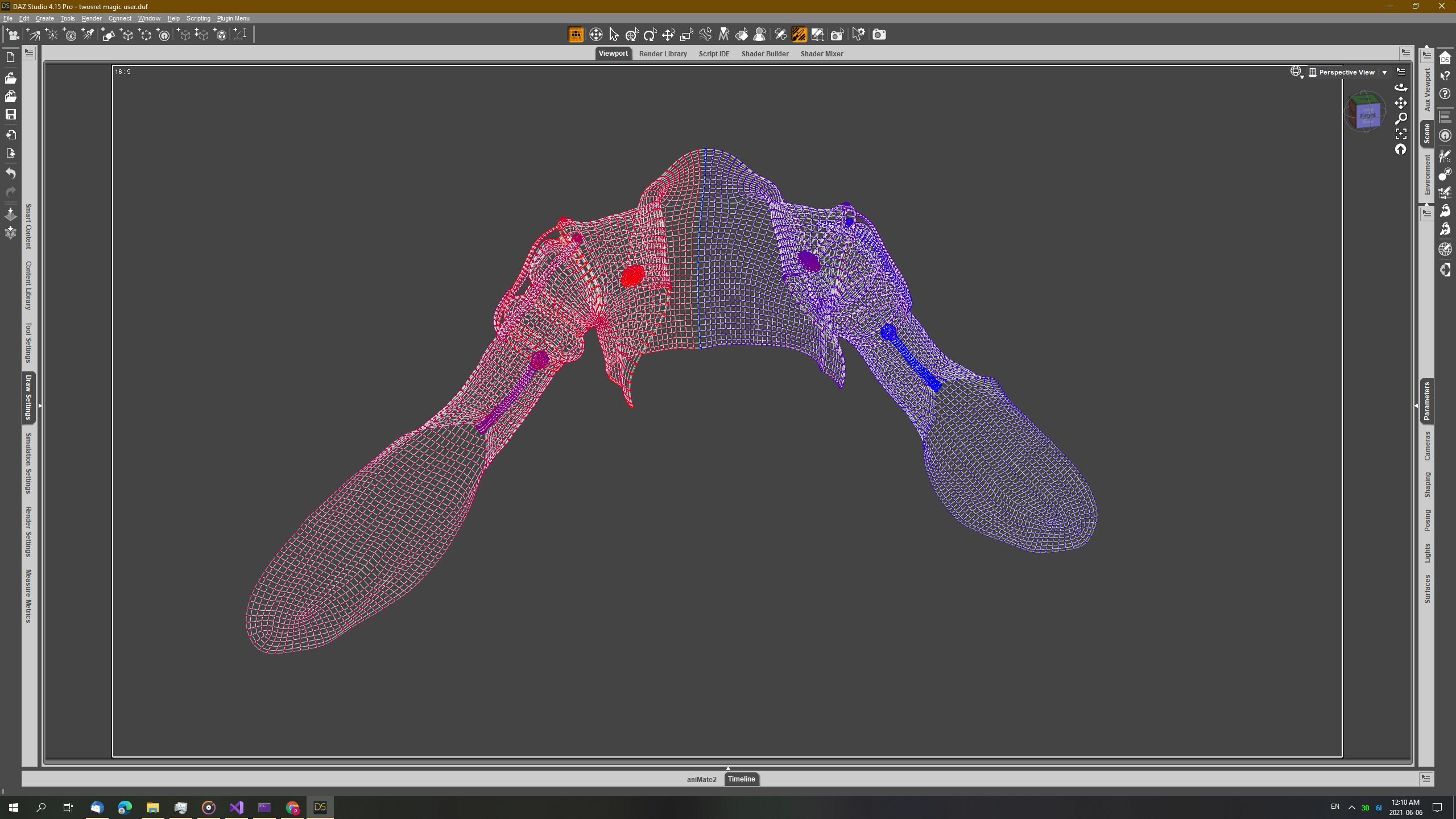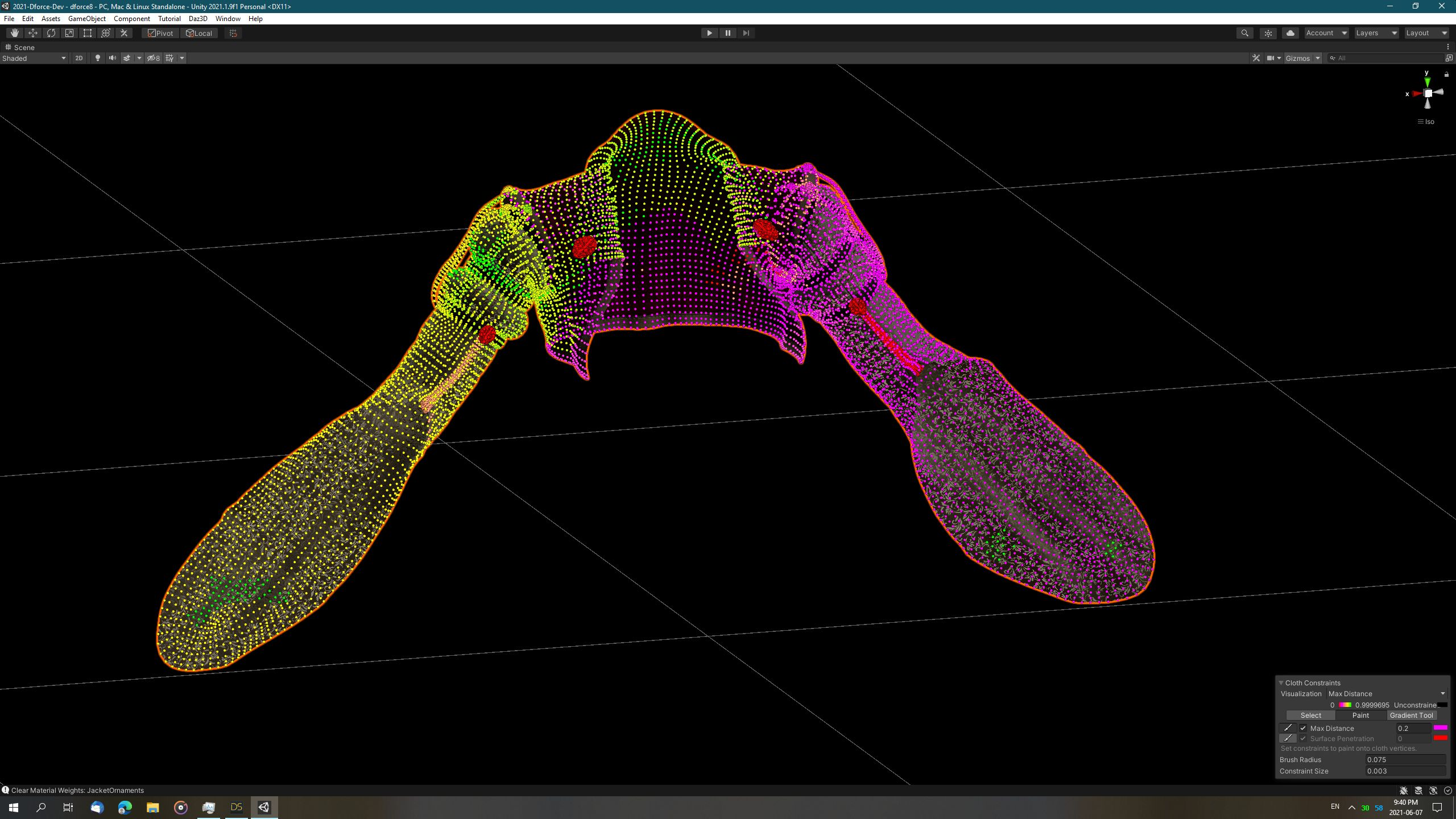Exporting (dForce) Weightmaps: Vertex Order?

Hi.  I've made some progress with exporting dForce clothing to Unity.  The basic dForce simulation parameters including per-Surface simulation data are being successfully read and exported to corresponing Unity Cloth Physics paramters.  However, I need help trying to export the Weightmap Data in the correct Vertex Order.  The vertex ordering in the Unity weightmap is very simple: it appears to be the same vertex ordering as what is stored in the mesh, with duplicate vertices removed in a sequential pattern (first equivalent vertex marked as unique, subsequent equivalent vertices marked as duplicate and removed).  This results in submeshes being "vertex-packed" and arranged in the same order as they appear in file.  I was hoping that the Daz Studio weightmap vertex ordering would be the same, but it appears that the vertex order is actually in some kind of binary tree or other optimized data structure:

When I write a gradient pattern with sequential values from minimum to maximum in Unity, this is what I get:The vertex weights are evenly distributed symmetrically, grouped by the submesh/material to which the vertex belongs.

When I write the same gradient pattern to Daz Studio's weightmaps, this is what I get:The vertex weights are split down the midline, but rather than being a smooth gradient from right to left, the weights appear to be distributed with an almost back-and-forth staggered arrangement with a very sharp contrast at certain points (see the midline).  To me, this looks almost like the distribution pattern from the array representation of a binary heap.  Obviously, this is not sorted by submesh/material group and the resulting export of the above gradient into Unity looks like this:From a programming standpoint, it seems logical to me that Daz Studio would be storing the weightmaps in the same vertex order that it stores the vertices in its internal mesh data / vertex buffers.  And it would be equally logical, that these vertex buffers would be sorted into some sort of array representation of a binary heap/tree for efficient geometry operations.  Can anyone shed light on exactly what the vertex ordering for the internal vertex buffers and corresponding weightmaps are?  Thanks much!

BTW, the latest source code for the dForce to Unity export functions can be found here: https://github.com/danielbui78/DazToRuntime/tree/dforce-experimental

The main thread for the Unofficial Daz to Unity Bridge (including dForce export) can be found here: https://www.daz3d.com/forums/discussion/494626/unofficial-updates-for-daztounity-bridge-urp-built-in-pbrskin-anims-dforce

Post edited by danielbui78 on

• From a programming standpoint, it seems logical to me that Daz Studio would be storing the weightmaps in the same vertex order that it stores the vertices in its internal mesh data / vertex buffers.  And it would be equally logical, that these vertex buffers would be sorted into some sort of array representation of a binary heap/tree for efficient geometry operations.  Can anyone shed light on exactly what the vertex ordering for the internal vertex buffers and corresponding weightmaps are?  Thanks much!

I think I have worked out a potential road map towards a solution (hopefully).  If my previous assumption that the weightmap vertex order is the same as the internal vertex order for all geometry, then I should be able to find the correct mapping of weight map value to vertex order by just starting with the first vertex of the first facet of the first facet material group of my target geometry object.  Then use the DzFacet::m_vertIdx lookup table to find the correct index into the geometry's vertex array.  And if I am hopefully correct in my above assumption, that will also be the index for that vertex in the weightmap data array.... fingers crossed.  Oh, and then I would also create a new assumption that the FbxExporter is simplistic enough that it exports all vertices sequentially in the vertex->facet->facet group/material group->geometry order used by Daz internal memory structures.  So, I would just follow that pattern and step through all vertices in that order, grab the (hopefully) correct index into the weightmap data array and then output it into a new array for use by Unity.

Update:

Well, this is embarrassing: the above algorithm is exactly what I implemented and committed to Github on June 7.  Usually it takes at least 6 months before I start forgetting large chunks of code and attempt to implement something that I've already implemented.  This is the literal embodiment of "those who forget the past are doomed to repeat it", LOL.

Unfortunately, my assumption has to be that this algorithm did not work if I still posted the original request for help 15 days later.  Seriously, if anyone has ANY ideas, suggestions or tips for me to solve this problem I would extremely appreciate it.  Thanks.

Post edited by danielbui78 on
• I'm not quite sure what you are doing, but the weight map is using the vertex list; the facets then grab vertices but the order of facets does not have to match the order of vertices, and of course each vertex is likely to belong to more than one facet (unless it's on a corner).

• Richard Haseltine said:

I'm not quite sure what you are doing, but the weight map is using the vertex list; the facets then grab vertices but the order of facets does not have to match the order of vertices, and of course each vertex is likely to belong to more than one facet (unless it's on a corner).

Thanks so much for the feedback!  That gives me hope that I've actually been on the right path regarding the weight map value to vertex indexes, or at least looking in the correct general direction.  I will try to make several dforce weight map demos to prove to myself that I'm looking at the correct weight map value / vertex index.  If that's successfull, then my next step would be to figure out if I'm conforming to the correct order of facets being outputted by the FbxExporter.

• https://banexdevblog.wordpress.com/2014/06/23/a-quick-tutorial-about-the-fbx-ascii-format/ seems to indicate that the FBX fromat has a vertex list for each object, those should - as far as I know - be in the order used internally in DS. The list of facets follows that section, giving the index of the vertices it contains (with the last one being XORed with -1 for facets with more than three vertices). Sorry if this is totally beside the point.

• Idk if this wil help but Ive been working on a script that prints all of the dforce maps.  The weights are in the same order as the vertex indices.

(function(){
var node=Scene.getPrimarySelection();
var object=node.getObject();
var n=object.getNumModifiers();
var mod;
var weightMap;
for(var i=0;i<n;i++){
mod=object.getModifier(i);
if(mod.getName().find("dForce Simulation")>-1){
pm(mod);
break;
}
}

var s="";
var wm=mod.getInfluenceWeights();
if(wm){
s="var influenceW="+getWeightString(wm);
}

print(s);
wm=mod.getVelocitySmoothingWeights();
if(wm){
s="var velocityW="+getWeightString(wm);
print(s);
}

wm=mod.getMassDensityWeights();
if(wm){
s="var densityW="+getWeightString(wm);
print(s);
}

wm=mod.getStretchStiffnessWeights();
if(wm){
s="var stretchW="+getWeightString(wm);
print(s);
}

wm=mod.getSurfaceSmoothingWeights()
if(wm){
s="var smoothW="+getWeightString(wm);
print(s);
}

wm=mod.getShearStiffnessWeights();
if(wm){
s="var shearW="+getWeightString(wm);
print(s);
}

wm=mod.getBendStiffnessWeights();
if(wm){
s="var bendW="+getWeightString(wm);
print(s);
}

wm=mod.getBucklingRatioWeights();
if(wm){
s="var buckRW="+getWeightString(wm);
print(s);
}

wm=mod.getBucklingStiffnessWeights();
if(wm){
s="var buckSW="+getWeightString(wm);
print(s);
}

acceptUndo("Script");
})();

function getWeightString(wm){
var s="[";
var n;
for(var i=0;i<wm.getNumWeights();i++){
if(i>0)s+=",";
n=wm.getFloatWeight(i)
s+=toPrecision(n,4);
}
s+="];";
return s;
}

function toPrecision(n,p){
if(n<=1.0/(10*p)){
return "0";
}
if(n>=1-(1.0/(10*p))){
return "1";
}
return n.toPrecision(p);
}

Post edited by gamplod on